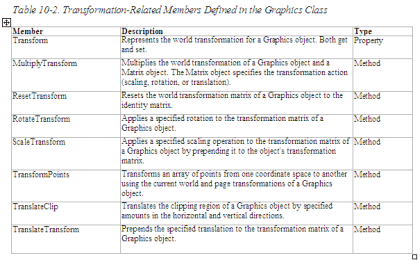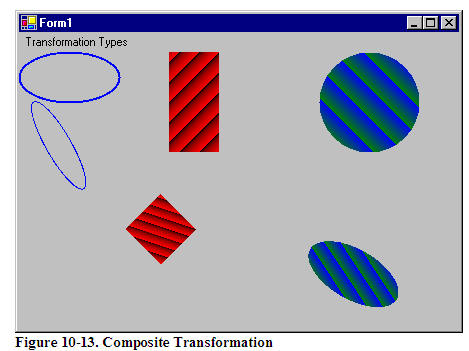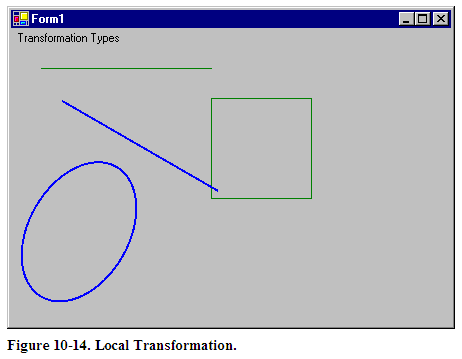# The Graphics Class and Transformations

In Chapter 3 we saw that the Graphics class provides some transformation-related members. Before we move to other transformation-related classes, let's review the transformation functionality defined in the Graphics class, as described in Table 10.2. We will see how to use these members in the examples throughout this chapter.The Transform property of the Graphics class represents the world transformation of a Graphics object. It is applied to all items of the object. For example, if you have a rectangle, an ellipse, and a line and set the Transform property of the Graphics object, it will be applied to all three items. The Transform property is a Matrix object. The following code snippet creates a Matrix object and sets the Transform property:

Matrix X = new Matrix();
X.Scale(2, 2, MatrixOrder.Append);
g.Transform = X;

The transformation methods provided by the Graphics class are MultiplyTransform, ResetTransform, RotateTransform, ScaleTransform, TransformPoints, TranslateClip, and TranslateTransform. The MultiplyTransform method multiplies a transformation matrix by the world transformation coordinates of a Graphics object. It takes an argument of Matrix type. The second argument, which specifies the order of multiplication operation, is optional. The following code snippet creates a Matrix object with the Translate transformation. The MultiplyTransform method multiplies the Matrix object by the world coordinates of the Graphics object, translating all graphics items drawn by the Graphics object.

Matrix X = new Matrix();
X. Translate(200.0F, 100.0F);
g.MultiplyTransform(X, MatrixOrder.Append);

RotateTransform rotates the world transform by a specified angle. This method takes a floating point argument, which represents the rotation angle, and an optional second argument of MatrixOrder. The following code snippet rotates the world transformation of the Graphics object by 45 degrees:

g.RotateTransform(45.0F, MatrixOrder.Append);

The ScaleTransform method scales the world transformation in the specified x- and y-directions. The first and second arguments of this method are x- and y-direction scaling factors, and the third optional argument is MatrixOrder. The following code snippet scales the world transformation by 2 in the x-direction and by 3 in the y-direction:

g.ScaleTransform(2.0F, 3.0F, MatrixOrder.Append);

The TranslateClip method translates the clipping region in the horizontal and vertical directions. The first argument of this method represents the translation in the x-direction, and the second argument represents the translation in the y-direction:
e.Graphics.TranslateClip(20.0f, 10.0f);
The TranslateTransform method translates the world transformation by the specified x- and y-values and takes an optional third argument of MatrixOrder:

g.TranslateTransform(100.0F, 0.0F, MatrixOrder.Append);

We will use all of these methods in our examples.

10.5 Global, Local, and Composite Transformations

Transformations can be divided into two categories based on their scope: global and local. In addition, there are composite transformations. A global transformation is applicable to all items of a Graphics object. The Transform property of the Graphics class is used to set global transformations.

A composite transformation is a sequence of transformations. For example, scaling followed by translation and rotation is a composite translation. The MultiplyTransform, RotateTransform, ScaleTransform, and TranslateTransform methods are used to generate composite transformations.

Listing 10.14 draws two ellipses and a rectangle, then calls ScaleTransform, TranslateTransform, and RotateTransform (a composite transformation). The items are drawn again after the composite transformation.

Listing 10.14: Applying a composite transformation

private void GlobalTransformation_Click(object sender,System.EventArgs e)
{
// Create a Graphics object
Graphics g = this
.CreateGraphics();
g.Clear(
this
.BackColor);
// Create a blue pen with width of 2
Pen bluePen = new
Pen(Color.Blue, 2);
Point pt1 =
new
Point(10, 10);
Point pt2 =
new
Point(20, 20);
Color [] lnColors = {Color.Black, Color.Red};
Rectangle rect1 =
new
Rectangle(10, 10, 15, 15);
// Create two linear gradient brushes
new
(pt1, pt2, Color.Red, Color.Green);
// Set linear colors
lgBrush.LinearColors = lnColors;
// Set gamma correction
lgBrush.GammaCorrection = true
;
// Fill and draw rectangle and ellipses
g.FillRectangle(lgBrush, 150, 0, 50, 100);
g.DrawEllipse(bluePen, 0, 0, 100, 50);
g.FillEllipse(lgBrush1, 300, 0, 100, 100);
// Apply scale transformation
g.ScaleTransform(1, 0.5f);
// Apply translate transformation
g.TranslateTransform(50, 0, MatrixOrder.Append);
// Apply rotate transformation
g.RotateTransform(30.0f, MatrixOrder.Append);
// Fill ellipse
g.FillEllipse(lgBrush1, 300, 0, 100, 100);
// Rotate again
g.RotateTransform(15.0f, MatrixOrder.Append);
// Fill rectangle
g.FillRectangle(lgBrush, 150, 0, 50, 100);
// Rotate again
g.RotateTransform(15.0f, MatrixOrder.Append);
// Draw ellipse
g.DrawEllipse(bluePen, 0, 0, 100, 50);
// Dispose of objects
lgBrush1.Dispose();
lgBrush.Dispose();
bluePen.Dispose();
g.Dispose();

Figure 10.15 shows the output from Listing 10.14.A local transformation is applicable to only a specific item of a Graphics object. The best example of local transformation is transforming a graphics path. The Translate method of the GraphicsPath class translates only the items of a graphics path. Listing 10.15 translates a graphics path. We create a Matrix object and apply rotate and translate transformations to it.

Listing 10.15: Translating graphics path items

private void LocalTransformation_Click(object sender, System.EventArgs e)
{
// Create a Graphics object
Graphics g = this
.CreateGraphics();
g.Clear(
this
.BackColor);
// Create a GraphicsPath object
GraphicsPath path = new
GraphicsPath();
// Add an ellipse and a line to the
// graphics path
// Create a blue pen with a width of 2
Pen bluePen = new
Pen(Color.Blue, 2);
// Create a Matrix object
Matrix X = new
Matrix();
// Rotate 30 degrees
X.Rotate(30);
// Translate with 50 offset in x direction
X.Translate(50.0f, 0);
// Apply transformation on the path
path.Transform(X);
// Draw a rectangle, a line, and the path
g.DrawRectangle(Pens.Green, 200, 50, 100, 100);
g.DrawLine(Pens.Green, 30, 20, 200, 20);
g.DrawPath(bluePen, path);
// Dispose of objects
bluePen.Dispose();
path.Dispose();
g.Dispose();
}

Figure 10.16 shows the output from Listing 10.15. The transformation affects only graphics path items (the ellipse and the blue [dark] line).